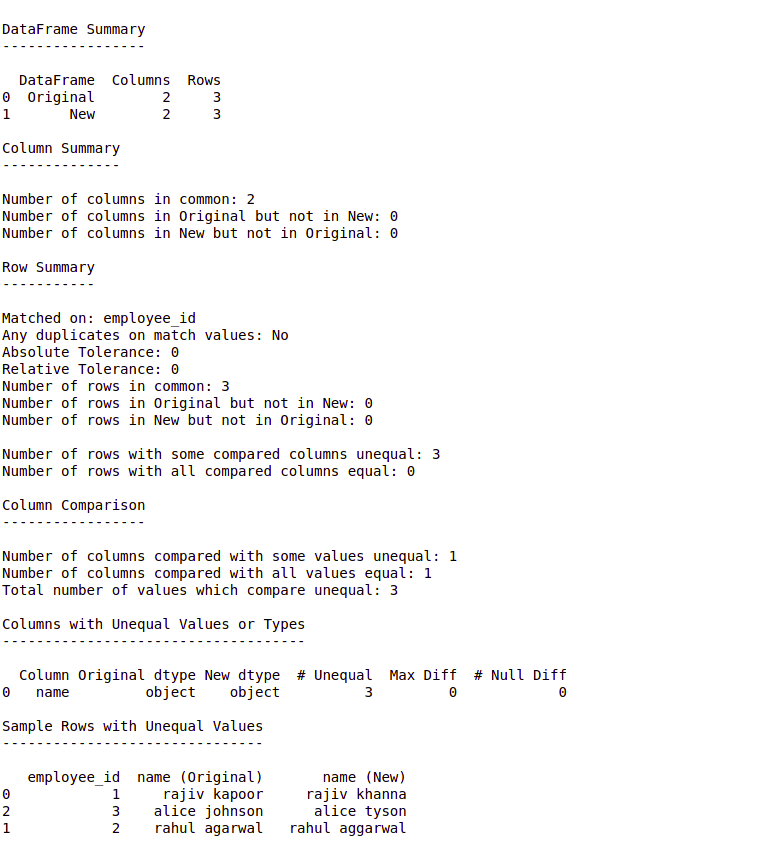# Compare Pandas Dataframes using DataComPy

It’s well known that Python is a multi-paradigm, general-purpose language that is widely used for data analytics because of its extensive library support and an active community. The most commonly known methods to compare two Pandas dataframes using python are:

These methods are widely in use by seasoned and new developers but what if we require a report to find all of the matching/mismatching columns & rows? Here’s when the DataComPy library comes into the picture.

DataComPy is a Pandas library open-sourced by capitalone. It was started with an aim to replace PROC COMPARE for Pandas data frames. It takes two dataframes as input and gives us a human-readable report containing statistics that lets us know the similarities and dissimilarities between the two dataframes.

Install via pip3:

```pip3 install datacompy
```

Example:

 `from` `io ``import` `StringIO ` `import` `pandas as pd ` `import` `datacompy ` `  `  `     `  `data1 ``=` `"""employee_id, name ` `1, rajiv kapoor ` `2, rahul agarwal ` `3, alice johnson ` `"""` `  `  `data2 ``=` `"""employee_id, name ` `1, rajiv khanna ` `2, rahul aggarwal ` `3, alice tyson ` `"""` `  `  `df1 ``=` `pd.read_csv(StringIO(data1)) ` `df2 ``=` `pd.read_csv(StringIO(data2)) ` `  `  `compare ``=` `datacompy.Compare( ` `    ``df1, ` `    ``df2, ` `     `  `    ``# You can also specify a list ` `    ``# of columns ` `    ``join_columns ``=` `'employee_id'``,  ` `     `  `    ``# Optional, defaults to 0 ` `    ``abs_tol ``=` `0``, ` `     `  `    ``# Optional, defaults to 0 ` `    ``rel_tol ``=` `0``,  ` `     `  `    ``# Optional, defaults to 'df1' ` `    ``df1_name ``=` `'Original'``, ` `     `  `    ``# Optional, defaults to 'df2' ` `    ``df2_name ``=` `'New'`  `    ``) ` ` `  `# if ignore_exra_columns=True,  ` `# the function won't return False ` `# in case of non-overlapping  ` `# column names ` `compare.matches(ignore_extra_columns ``=` `False``)    ` `  `  `# This method prints out a human-readable  ` `# report summarizing and sampling  ` `# differences ` `print``(compare.report()) `

Output:Explanation:

• In the above example, we are joining the two data frames on a matching column. We can also pass: `on_index = True` instead of “join_columns” to join on the index instead.
• ` Compare.matches()` is a Boolean function. It returns True if there’s a match, else it returns False.
• DataComPy by default returns True only if there’s a 100% match. We can tweak this by setting the values of abs_tol & rel_tol to non-zero, which empowers us to specify an amount of deviation between numeric values that can be tolerated. They stand for absolute tolerance and relative tolerance respectively.
• We can see from the above example that DataComPy is a really powerful library & it is extremely helpful in cases when we have to generate a comparison report of 2 dataframes.

My Personal Notes arrow_drop_upCheck out this Author's contributed articles.

If you like GeeksforGeeks and would like to contribute, you can also write an article using contribute.geeksforgeeks.org or mail your article to contribute@geeksforgeeks.org. See your article appearing on the GeeksforGeeks main page and help other Geeks.

Please Improve this article if you find anything incorrect by clicking on the "Improve Article" button below.